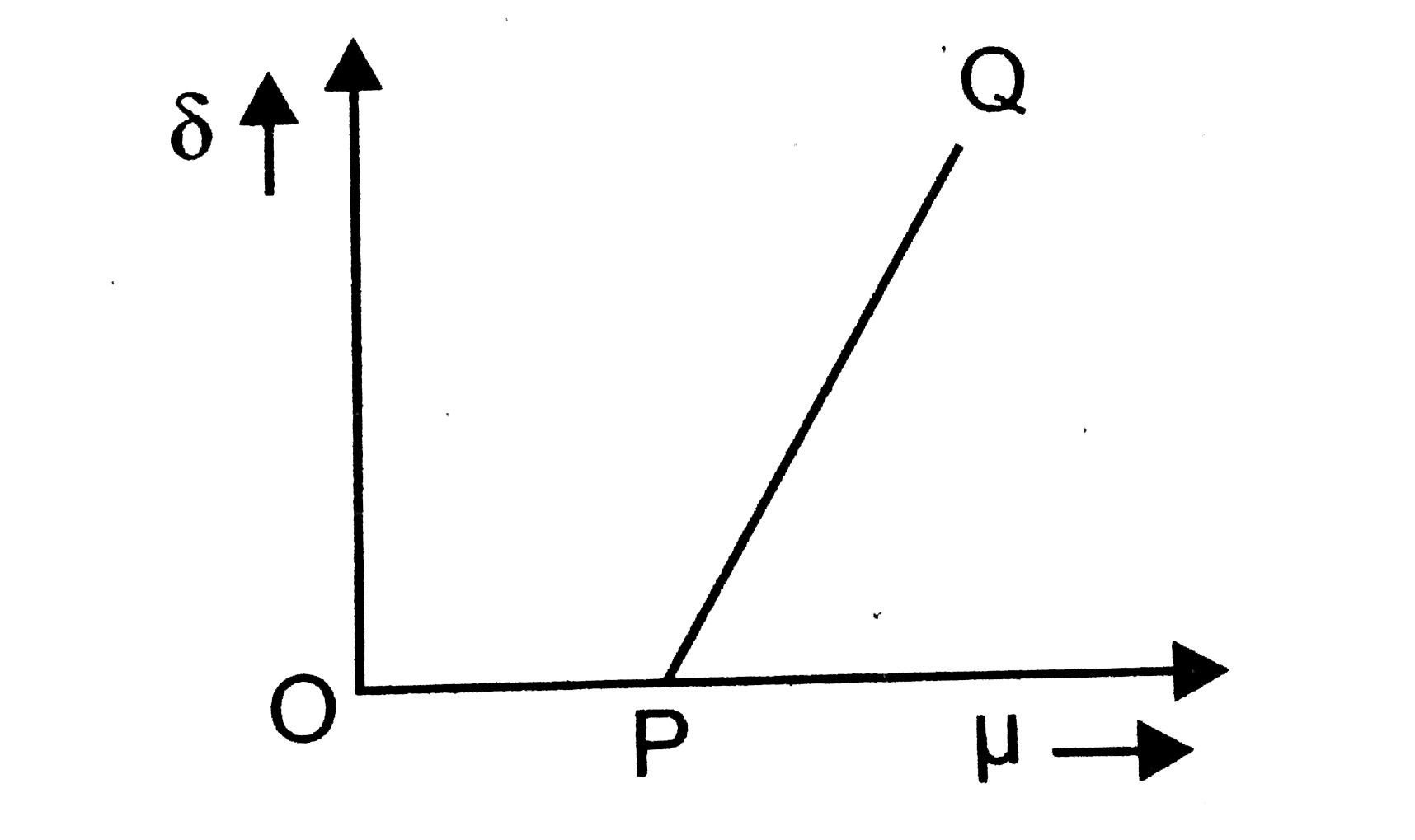# For a small angled prism, angle of prism A of minimum deviation(delta) varies with the refractive index of the prism as shown in the graph

39 views
in Physics
closed
For a small angled prism, angle of prism A of minimum deviation(delta) varies with the refractive index of the prism as shown in the graphA. Point P corresponds to mu=1//2
B. Slope of the line PQ=A//2
C. Slope=A
D. None of the above statements is true

by (84.1k points)
selected

delta=(mu-1)Aimpliesy=(x-1)A=xA-A
Comparing with y=mx+C
Slope: m=A